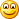# Project Euler – Problem 74 Solution

#### Problem

The number 145 is well known for the property that the sum of the factorial of its digits is equal to 145:

1! + 4! + 5! = 1 + 24 + 120 = 145

Perhaps less well known is 169, in that it produces the longest chain of numbers that link back to 169; it turns out that there are only three such loops that exist:

1693636011454169

87145361871

87245362872

It is not difficult to prove that EVERY starting number will eventually get stuck in a loop. For example,

693636001454169363601 (1454)

784536087145361 (871)

540145 (145)

Starting with 69 produces a chain of five non-repeating terms, but the longest non-repeating chain with a starting number below one million is sixty terms.

How many chains, with a starting number below one million, contain exactly sixty non-repeating terms?

#### Solution

The solution I have here caches the previous results to avoid duplicated work, this complicates the code slightly but it should still be fairly easy to follow. The key thing here is the recursive function processChainRec which recursively generate the next number in the chain until it hits one of two conditions:

1. you hit a number already in the chain (hence the end of the non-repeating chain)

2. you hit a number whose non-repeating chain length has been cached

In the first case, suppose you have [69, 363600, 1454, 169, 363601] in the list already, and then the next number is 1454, which is in the list with index 2. At this point, you can work out the non-repeating chain length for 69, 363600 and 1454:

69 : list.length – index = 5 – 0 = 5

363600 : list.length – index = 5 – 1 = 4

1454 : list.length – index = 5 – 2 = 3

Hit three eggs with one stone? AwesomeFor the second case, suppose you had already come across 1454 before and have already calculated its non-repeating chain length, now you have [69, 363600] in the list and 1454 pop outs of the hat. So a quick look up in the cached data tells you that 1454 has a non-repeating chain of 3 terms, so to apply that knowledge to 69 and 363600:

69 : list.length – index + 3 = 2 – 0 + 3 = 5

363600 : list.length – index + 3 = 2 – 1 + 3 = 4

Voila!